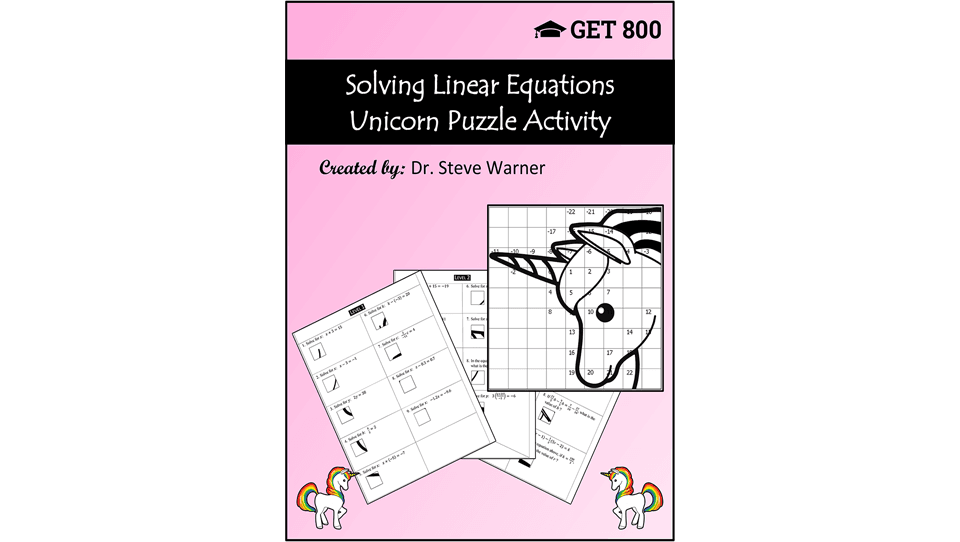# Blog

##### Solving Linear Equations - Unicorn Puzzle ActivityThis one is for the math teachers. Sticking with the unicorn theme, I just made a math activity for those of you teaching algebra.

This activity consists of 5 levels of problems all involving solving linear equations. Each level consists of 9 problems. Each problem comes with a puzzle piece, and the solution to the problem tells you where to place the piece. I prefer to have the students simply draw the piece in the appropriate place in the answer grid, but you can also have them paste the piece in. The final result is a unicorn head, as displayed on the cover above.

Problems start out with very simple equations and proceed to more difficult word problems that are consistent with the types of problems students will see on standardized tests such as the SAT. In fact, students that can solve all of the problems given in this activity will have no trouble with SAT math problems about solving linear equations.

The cost for this activity is \$3.99, and it is available in my teachers pay teachers store here: Solving Linear Equations - Unicorn Puzzle Activity

The levels of the problems are as follows:

Level 1: One-step equations
Level 2: Two-step equations
Level 3: Distributive property and combining like terms
Level 4: Variable on both sides of the equation
Level 5: Word problems

Complete solutions are included for all 45 problems.

If you have any questions, feel free to contact me at steve@SATPrepGet800.com

And as always, thanks for your support!